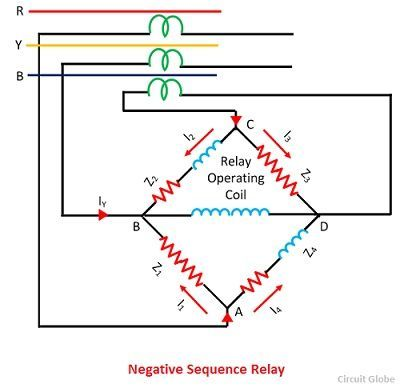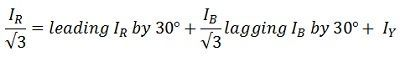# Negative Sequence Relay

Definition: A relay which protects the electrical system from negative sequence component is called a negative sequence relay or unbalance phase relay. The negative sequence relay protects the generator and motor from the unbalanced load which mainly occurs because of the phase-to-phase faults.

The negative sequence relay has a filter circuit which operates only for the negative sequence components. The relay always has a low current setting because the small magnitude overcurrent can cause dangerous situations. The negative sequence relay has earthing which protects them from phase to earth fault but not from phase to phase fault. The phase to phase fault mainly occurs because of the negative sequence components.

The construction of the negative sequence relay is shown in the figure below. The Z1, Z2, Z3, and Z4 are the four impedance of the circuit which is connected in the form of the bridge. The impedance is energized by the current transformers. The relay operating coil is connected to the midpoint of the circuit as shown in the figure below.The Z1 and Z3 are purely resistive and the Z2 and Z4 are both resistive and inductive in nature. The impedance Z2 and Z4 are adjusted in such a manner that the current flowing through them is always lagging by an angle of 60º than those current which is flowing through Z1 and Z3. The current flowing through the junction A is split into two parts i.e. I1 and I4. The I4 lagging by an angle of 60º regards I1.Similarly, current from phase B split at junction C into two equal components I3 and I2, I2 lagging behind I3 by 60º.The current I4 lags by an angle of 30º to the I1. Similarly, I2 lags by an angle of 30º concerning IB and I3 leads IB by 30º. The current passing through the junction B is equal to the sum of I1, I2, and IY.The flow of Positive Sequence Current – The phasor diagram of positive sequence components is shown in the figure below. When the load is in balanced conditions, then there is no negative sequence current. The current flows through the relay is given by the equationSo the relay remains operative for a balanced system.

The flow of Negative Sequence Current – The figure above shows that the current I1 and I2 are equal. Thus, they cancel each other. The current IY flows through the operating coils of the relay. The current setting value of the relay is kept less than the normal full load rating current because the small overload current can cause the serious conditions.

The flow of Zero Sequence Current  – The current I1 and I2 are displaced from each other by an angle of 60º. The resultant of the current is in phase with the current IY. The total current twice of the zero sequence current flows through the operating coil of the relay. The relay can be inoperative by connecting the CTs in the delta. In delta connection no zero sequence current flows through the relay.

###Induction type Negative Sequence Relay

The construction of induction type negative phase sequence relay is similar as that of an induction type overcurrent relay. This relay consists of a metallic disc usually made up of an aluminium coil, and this is rotating between two electromagnets the upper and the lower electromagnets.

The upper electromagnet has two winding, the primary winding of the upper electromagnet is connected to the secondary of the CT connected in the line to be protected. The secondary winding of the upper electromagnet is connected in series with the windings on the lower electromagnet

The primary winding of the relay has three terminals because of the centre tapping. The phase R energized the upper half of the relay by the help of CTs and auxiliary transformer while the lower half is energized by the phase Y. The auxiliary transformer is so adjusted that their output is lagged by an angle of 120º instead of 180º.

The operation for Positive Sequence Currents – The current IR and IY flow through the primary windings of the relay. The current flows in the opposite direction. The current I’R and I’Yare equal in magnitude. The balanced current kept the relay inoperative.

The operation for Negative Sequence currents – The negative sequence current I flow in the primary winding of the relay because of the fault current.The relay starts operating when the magnitude of the fault current is more than that of the relay setting.